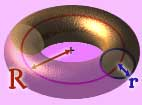# Thread: General equation question - big problems...

1. Hey all, if there is a high up math whiz maybe you can help me out here on this one

I'm kind of not a HUGE math fan but am wondering on a couple points...(hope this isn't like a RANDOM question)

My question is actually fairly simple: can equations and the like be configured (set) so that they might reflect something from higher than 3rd dimension? i.e 4th 5th and higher?

not sure if this is accurate or not, however, is there not more than 3 known dimensions in reality? i'm not asking this in physics forum because i'm asking about the math side of this!

for example, could someone write an equation that demonstrates what an object would look like in the 4th dimension, or similar? Certainly I'd like someone to back up that if it's indeed a fact.

unfortunately, I went all the way to Algebra only as HS grad! no calc, trig nothing.if someone could point this out for me I'll give you a slap on the back and possibly...say...\$300 monopoly dollars. :P

Tom Anderson2.

3. It's really simple, just keep adding independent variables and the dimension keeps going up.

A circle in 2D is defined by sqrt(x^2 + y^2) = 1
A sphere in 3D is defined by sqrt(x^2 + y^2 + z^2) = 1
A hypersphere in 4D is defined by sqrt(w^2 + x^2 + y^2 + z^2) = 1 (not that w is a spatial dimension, not a temporal one)
In fact, any such equation (or system of equations) in 4 (independent) variables defines some shape in 4-space. For example, sqrt(w^2 + x^2) = 1 and sqrt(y^2 + z^2) = 1 defines a 4D torus (if I'm not mistaken).

You can keep going too. A sphere in 26D would be sqrt(a^2 + b^2 + ... + y^2 + z^2) = 1. (If you go past 26, you'll have to use subscripts to keep up with the variables though.)

One thing. I said independent variables. In sqrt(w^2 + x^2) = 1 and sqrt(y^2 + z^2) = 1, you have 4 variables in 2 equations, so you really only have 2 independent variables. So this equation actually describes a 4D embedding of the surface of a torus (which is 2D). The equation for the 4D hypersphere above actually gives its 3D shell. If you want the whole hypersphere, you can just make it an inequallity.4.Originally Posted by MagiMaster
For example, sqrt(w^2 + x^2) = 1 and sqrt(y^2 + z^2) = 1 defines a 4D torus (if I'm not mistaken).
Can you explain why it is a torus? My guess is it is more like a cylinder, but with two rotational axes.5. Thanks!

Cheers
Tom A.6.Originally Posted by temurOriginally Posted by MagiMaster
For example, sqrt(w^2 + x^2) = 1 and sqrt(y^2 + z^2) = 1 defines a 4D torus (if I'm not mistaken).
Can you explain why it is a torus? My guess is it is more like a cylinder, but with two rotational axes.
It's more like a circle crossed with a circle (Euclidian cross product). I can see that it's a torus in my head, but I'm having trouble explaining it. Like any torus, it's a cylinder with the ends joined together. (Actually, I'm not 100% sure what I see in my head is what those equations describe, but I'm fairly certain.) Maybe someone else will be able to explain it better.7. Okay, the idea is this - if you take a circle and sweep it along the circumference of a larger circle so that its radius stays on the circumference of that larger circle you can get a torus (i hope you can picture what i am saying).Now lets consider using these two circles to describe a point on that torus. Well we need to specify where we are on the circumference of the larger circle and to do that we can give two numbers u and v such that u<sup>2</sup> + v<sup>2</sup> = R<sup>2</sup> where R is the radius of the larger circle. Then we need to say where we are on the smaller circle which swept out the torus and to do that we need to give two numbers x, y such that x<sup>2</sup> + y<sup>2</sup> = r<sup>2</sup> where r is the radius of the smaller circle. Now if you divide each equation by R<sup>2</sup> and r<sup>2</sup> respectively you get a set of four numbers (x', y', u', v') such that (x')<sup>2</sup> + (y')<sup>2</sup> = 1 and (u')<sup>2</sup> + (v')<sup>2</sup> = 1.

So what we get here is a torus (not a 4D one by the way, just a torus in 4 space). I hope that helps, if not please shout.

Oh and a correction to Magi masters other posts - ignore all the square roots!8. Man... I'm not good at explaining stuff.I was thinking 'distance from point equals one.'9. hi magi the square roots are not wrong, they are just not needed so why keep them? rrBookmarks
 Posting Permissions
 You may not post new threads You may not post replies You may not post attachments You may not edit your posts   BB code is On Smilies are On [IMG] code is On [VIDEO] code is On HTML code is Off Trackbacks are Off Pingbacks are Off Refbacks are On Terms of Use Agreement